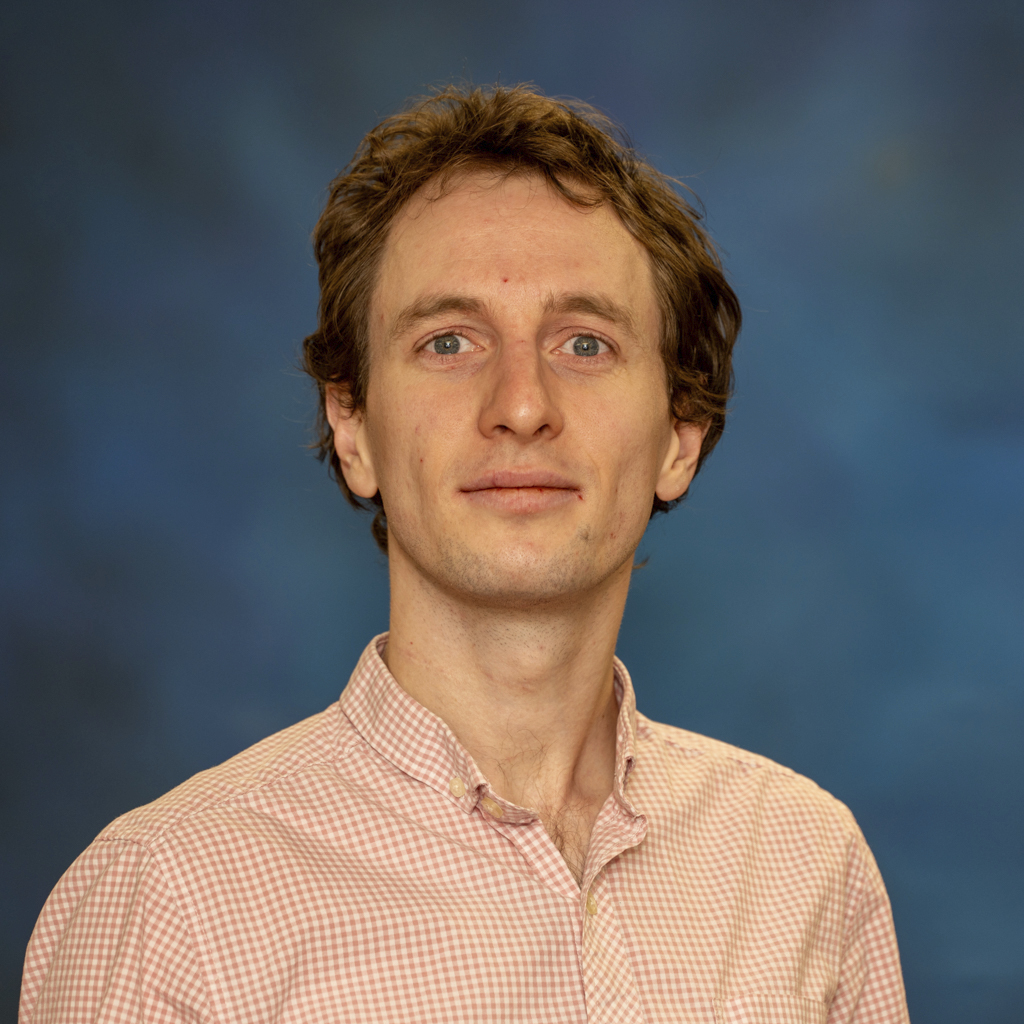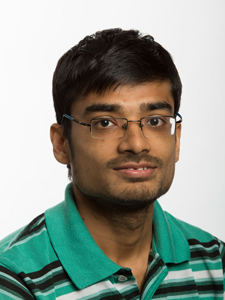COSAM » Departments » Mathematics & Statistics » Research » Seminars » Topology

# Topology

DMS Topology Seminar
Apr 26, 2023 01:00 PM
ZOOM

Title: On Lindelof scattered subspaces of nice $$sigma$$-products

Abstract: We will show that there exists an Eberlein compact space $$K$$ such that some Lindelöf subspace of $$K$$ fails to be a Lindelöf $$\Sigma$$-space. We also prove that any scattered Lindelöf subspace of a $$\sigma$$-product of first countable spaces is $$\sigma$$-compact. It is established that if $$X$$ is the $$G_\delta$$-modification of a scattered compact space, then $$ext(C_p(X)) = \omega$$.

DMS Topology Seminar
Mar 29, 2023 01:00 PM
224 Parker HallSpeaker: Ziqin Feng

Title: On Vietoris-Rips Complex of Finite Metric SpacesAbstract: I will discuss the homotopy type of the Vietoris-Rips complex of finite metric spaces. These are related to the independent complex of the Kneser graphs and VR complex on hypercube graphs.

DMS Topology Seminar
Mar 01, 2023 01:00 PM
224 Parker Hall and ZOOMSpeaker: Joseph Briggs Title: Infinitary DriskoAbstract: An elegant result from the 90's states that any filling of a 2𝑛 − 1 × 𝑛 array with distinct symbols in each column has a full transversal, namely a collection of 𝑛 cells from distinct rows and columns each with all entries distinct. The famous Ryser-Brualdi-Stein Conjecture from the 70’s suggests that for transversals of size 𝑛 − 1, such an 𝑛 × 𝑛 array suffices. This mysterious jump from 𝑛 to 2𝑛 − 1 columns remains elusive 30 years later, so we embark on a discussion of infinite variants in the hope of shedding some light.

DMS Topology Seminar
Feb 22, 2023 01:00 PM
224 Parker HallSpeaker: Brian Freidin Title: Free boundary minimal surfaces in the ballAbstract: Minimal surfaces are critical points for the area function on the space of submanifolds, say of R^n. In a bounded region V the free boundary problem asks for critical points of the area function on the space of submanifolds with boundary contained in the boundary of V. We will survey some results about what topologies can occur (classified by genus and number of boundary components in dimension 2) for free boundary minimal surfaces in the ball. Then we will discuss a construction for such surfaces by searching in the lower-dimensional space of G-invariant surfaces, for various groups G.

DMS Topology Seminar
Feb 15, 2023 01:00 PM
224 Parker HallSpeaker: Hannah Albert Title: Homology of configuration spaces of squares in a rectangleAbstract: We consider the configuration space of n unit squares sliding in a p by q rectangle.  In which degrees is its homology concentrated?  Squares in a rectangle serve as a model for molecules in a container.  Can we detect (approximately) whether the substance is a solid, liquid, or gas, using only the topology of the configuration space?  Even very basic questions about these configuration spaces tend to be unresolved, so there are many appealing directions for future research.

DMS Topology Seminar
Jan 27, 2023 01:00 PM
224 Parker HallSpeaker: Will Brian, University of North Carolina, Charlotte

Title: Large metric spaces and partitions of the real line into Borel setsAbstract: I will sketch a proof that, assuming $0^dagger$ does not exist, if there is a partition of $R$ into $ℵ_ω$ Borel sets, then there is also a partition of $R$ into $ℵ_{ω+1}$ Borel sets. (And the same is true for any singular cardinal of countable cofinality in place of $ℵ_ω$.) This contrasts starkly with the situation for cardinals with uncountable cofinality and their successors, where the spectrum of possible sizes of partitions of R into Borel sets can (via forcing) be made completely arbitrary. The proof of this fact for $ℵ_ω$ uses the structure of a certain complete metric space of weight $ℵ_ω$, and the existence of a particular partition of that space into Polish spaces.

DMS Topology Seminar
Jan 18, 2023 01:00 PM
224 Parker HallSpeaker: Arka Banerjee Title: Coarse cohomology of the complementAbstract: John Roe defined the notion of  Coarse cohomology of a metric space that measures the behavior at infinity of a space: more specifically, it measures the way in which uniformly large bounded sets fit together. In my talk I will give a brief introduction to this theory and define a new notion called "Coarse cohomology of the complement." Time permitting, I will discuss some related results and applications.

This talk is partly based on a joint work with Boris Okun.

DMS Topology Seminar
Nov 18, 2022 02:00 PM
224 Parker Hall and ZOOM

Speaker: Steven Clontz, University of South AlabamaTitle: Metrizability of Mahavier products indexed by partial orders

Abstract: Let $X$ be separable metrizable, and let $f\subseteq X^2$ be a non-trivial relation on $X$. For a given partial order $(P,\leq)$, the Mahavier product $M(X,f,P)\subseteq X^P$ (also known as a generalized inverse limit) collects functions such that $x(p)\in f(x(q))$ for all $p<q$. We will show that whenever $f$ satisfies condition $\Gamma$, $M(X,f,P)$ is separable metrizable if and only if $P$ is countable.

DMS Topology Seminar
Nov 11, 2022 02:00 PM
ZOOM

Speaker: Wlodek Kuperberg Title: Packing convex bodies in the plane and in space
DMS Topology Seminar
Nov 04, 2022 02:00 PM
224 Parker Hall and ZOOM